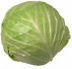### Fill in the blanks

1)Fill in the blanks:
 1151 � 17 = R 1149 � 13 = R
2)Weight of one cabbage is g.3) If Lisa's annual salary is Rs. 522696, her monthly salary will be Rs. .
 4) There are threes in 18 .
5)Fill in the blanks ( e.g. 7 � 2 = 3R1):
 538656 � 47 = R 93508 � 23 = R
6)Solve the following====

### Choose correct answer(s) from given choice

7)In division, the dividend can be specified in terms of divisor, quotient and remainder as:
 a. remainder X divisor + quotient b. quotient X divisor + remainder c. quotient X divisor d. quotient X remainder + divisor
8)= _____
 a. 8 b. 12 c. 14 d. 289
9)A number divided by one equals _________ .
 a. 0 b. the number itself c. 1 d. infinity
10)Kenneth paid Rs. 56 to the shopkeeper to buy 4 pens. What is the cost of each pen ?
 a. Rs. 11 b. Rs. 15 c. Rs. 14 d. Rs. 224